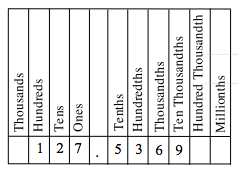### Home > MC2 > Chapter 1 > Lesson 1.2.4 > Problem1-92

1-92.

The value of a decimal becomes clearer when the place value is spoken or written as the number it names. For example, $0.1$ makes more sense if it is read as "one-tenth" rather than "zero point one."

1. Write the following numbers in words so that the place value can be identified.

$0.4$

$1.3$

$0.56$

$2.008$

2. Now reverse your thinking. Write the decimals that go with the following words.

thirty-five hundredths

three and two-tenths

six-hundredths

Make sure to pay attention to the last digit place of the decimal value. Use the chart to the right for guidance.

Look to see if there is a number to the left of the decimal; if so write it out. If there is no number to the left of the decimal, skip to step $3$.

Include 'and' for the decimal point if there is a number to the left of the decimal.

Write out the number to the right of the decimal up to the last digit. Write the place value of the last digit.$0.64 =$ sixty-four hundredths

With these steps, try writing each number in words.

Refer to part (a) for help with decimal places.

Consider the significance of the place value identified by the last word.

thirty-five hundredths $= 0.35$